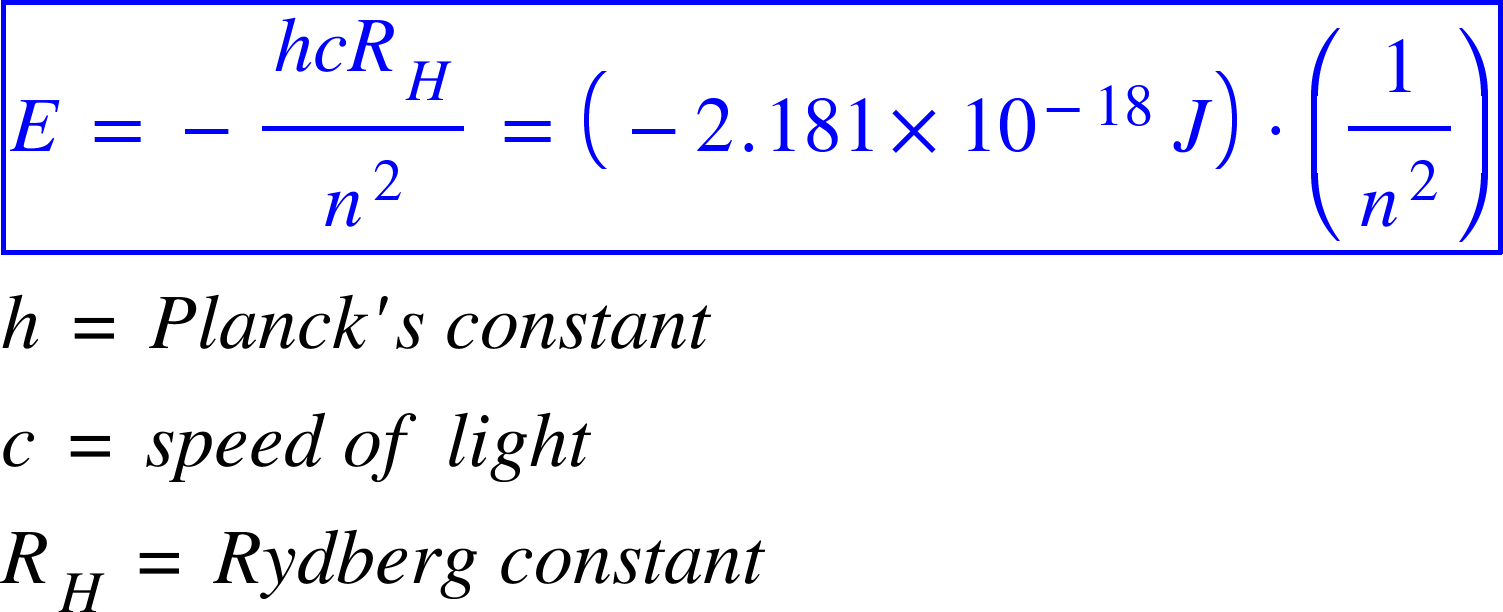# Problem: Using equation E= (hcRH)(1/n2) = (-2.1810-18J)(1/n2), calculate the energy of an electron in the hydrogen atom when n = 6.

🤓 Based on our data, we think this question is relevant for Professor Genereux's class at UCR.

###### FREE Expert Solution

The energy of an electron in a hydrogen atom is given by:The energy level or the shell, in which an electron revolves around the nucleus is termed as n.

In this case: We have n=6.###### Problem Details

Using equation E= (hcRH)(1/n2) = (-2.1810-18J)(1/n2), calculate the energy of an electron in the hydrogen atom when n = 6.